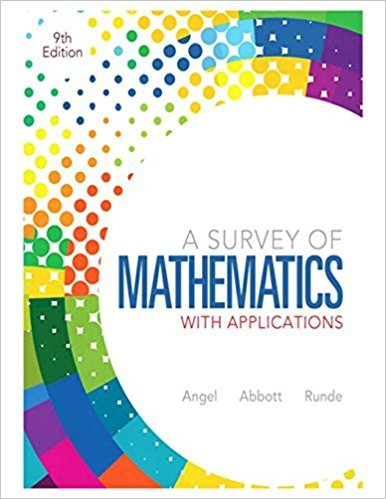×
×

# Solutions for Chapter 1.3: Critical Thinking Skills## Full solutions for A Survey of Mathematics with Applications | 9th Edition

ISBN: 9780321759665Solutions for Chapter 1.3: Critical Thinking Skills

Solutions for Chapter 1.3
4 5 0 366 Reviews
17
5
##### ISBN: 9780321759665

This textbook survival guide was created for the textbook: A Survey of Mathematics with Applications, edition: 9. Chapter 1.3: Critical Thinking Skills includes 68 full step-by-step solutions. This expansive textbook survival guide covers the following chapters and their solutions. A Survey of Mathematics with Applications was written by and is associated to the ISBN: 9780321759665. Since 68 problems in chapter 1.3: Critical Thinking Skills have been answered, more than 74234 students have viewed full step-by-step solutions from this chapter.

Key Math Terms and definitions covered in this textbook
• Cayley-Hamilton Theorem.

peA) = det(A - AI) has peA) = zero matrix.

• Cholesky factorization

A = CTC = (L.J]))(L.J]))T for positive definite A.

• Complex conjugate

z = a - ib for any complex number z = a + ib. Then zz = Iz12.

• Determinant IAI = det(A).

Defined by det I = 1, sign reversal for row exchange, and linearity in each row. Then IAI = 0 when A is singular. Also IABI = IAIIBI and

• Factorization

A = L U. If elimination takes A to U without row exchanges, then the lower triangular L with multipliers eij (and eii = 1) brings U back to A.

• Gauss-Jordan method.

Invert A by row operations on [A I] to reach [I A-I].

• Hankel matrix H.

Constant along each antidiagonal; hij depends on i + j.

• Hypercube matrix pl.

Row n + 1 counts corners, edges, faces, ... of a cube in Rn.

• Multiplicities AM and G M.

The algebraic multiplicity A M of A is the number of times A appears as a root of det(A - AI) = O. The geometric multiplicity GM is the number of independent eigenvectors for A (= dimension of the eigenspace).

• Multiplier eij.

The pivot row j is multiplied by eij and subtracted from row i to eliminate the i, j entry: eij = (entry to eliminate) / (jth pivot).

• Network.

A directed graph that has constants Cl, ... , Cm associated with the edges.

• Orthonormal vectors q 1 , ... , q n·

Dot products are q T q j = 0 if i =1= j and q T q i = 1. The matrix Q with these orthonormal columns has Q T Q = I. If m = n then Q T = Q -1 and q 1 ' ... , q n is an orthonormal basis for Rn : every v = L (v T q j )q j •

• Plane (or hyperplane) in Rn.

Vectors x with aT x = O. Plane is perpendicular to a =1= O.

• Pseudoinverse A+ (Moore-Penrose inverse).

The n by m matrix that "inverts" A from column space back to row space, with N(A+) = N(AT). A+ A and AA+ are the projection matrices onto the row space and column space. Rank(A +) = rank(A).

• Rayleigh quotient q (x) = X T Ax I x T x for symmetric A: Amin < q (x) < Amax.

Those extremes are reached at the eigenvectors x for Amin(A) and Amax(A).

• Right inverse A+.

If A has full row rank m, then A+ = AT(AAT)-l has AA+ = 1m.

• Simplex method for linear programming.

The minimum cost vector x * is found by moving from comer to lower cost comer along the edges of the feasible set (where the constraints Ax = b and x > 0 are satisfied). Minimum cost at a comer!

• Singular matrix A.

A square matrix that has no inverse: det(A) = o.

• Standard basis for Rn.

Columns of n by n identity matrix (written i ,j ,k in R3).

• Toeplitz matrix.

Constant down each diagonal = time-invariant (shift-invariant) filter.

×